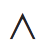Python是一种解释型、面向对象、动态数据类型的高级程序设计语言。 由于其语言的简洁、易读以“胶水般”的可扩展性，越来越多的童鞋选择使用Python进行科学计算。 Numpy是Python下的一个数值计算库，提供了多维数组，线性代数、傅里叶变换等众多数值计算函数。 如果使用Python+Numpy+Scipy(提供众多科学计算函数，基于Numpy)+matplotlib(一个强大的绘图库，绘图效果秒杀matlab！)，那么你几乎可以做在matlab下能做的任何事情。 网上有很多比较matlab与numpy的文章，他们的各自优缺点请自行谷歌。 在此提供一个matlab与numpy函数的对照表，从表中我们可以看出，numpy中的大多数函数的名称及使用方法与matlab中很相似，但是这并不意味这把程序从matlab移植到numpy中很容易， 其中的主要困难有：1、数组的引用方式（这是之后的重点）不同; 2、numpy中矩阵乘与数组乘的区别;3、数组的拼接方式不同，这些不同之处使得程序移植与把程序重新写一遍差不多。 matlab下有许多商业的工具箱，这些东西压根就没法移植。而如果你之前使用matlab积累了一些程序，那么把这些好不容易写成的程序都移植过去也不现实。 所以，保持两边各自的“画风”不变，仅仅交换数据，是matlab与numpy联合工作的最佳选择。 科学数据其实就是一大堆的数，其他的数据结构大都是用来辅助科学计算的，所以我们只需要实现两边多维数组数据的交换就可以了，交换其他的数据结构既费劲有没有太大的意义，好了，现在切入正题

## numpy与matlab 的数组引用差别

matlab中：Matlab


a = 1:120;
b = reshape(a, [2,3,4,5]);
b
b(:,:,1,1) =
1     3     5
2     4     6
b(:,:,2,1) =
7     9    11
8    10    12
.......
b(:,:,4,5) =
115   117   119
116   118   120


b是一个（2,3,4,5）的四维数组，它可以这么理解

[每一列有2个元素，每一矩阵有3列，每一页有4个矩阵，一共有5页]

（其中行，列大家都懂，页就像是几个矩阵叠起来写满一页）

matlab中比元素高一级的数组的单位是“列向量”，matlab储存数据是“按列”储存的。

matlab认为：“第几行”=“第几个列向量”， “第几列”=“列向量中的第几个元素”

reshape为（2,3,4,5）,matlab先把数据分成5页，每章有24个数据，再把一页的数据分为4个矩阵，每个矩阵有6个数据，这时第一个矩阵的数据应为1～6，这时候再把每一个矩阵的数据分为3行，所以每一列数据就变成有两个元素的列向量了。Matlab


[1,2,3;
4,5,6]


numpy中:python


a=np.array(range(1,121))
b=a.reshape((2,3,4,5))
b
array([[[[  1,   2,   3,   4,   5],
[  6,   7,   8,   9,  10],
[ 11,  12,  13,  14,  15],
[ 16,  17,  18,  19,  20]],
.........
[[101, 102, 103, 104, 105],
[106, 107, 108, 109, 110],
[111, 112, 113, 114, 115],
[116, 117, 118, 119, 120]]]])


numpy与C的数据储存方式相同，均是采用广义表的形式， 这一点上造成了numpy与C之间可以直接通过内存来传递数组（见ctypes）。

[一共有两章，每一章有3页，每一页有4行，每一行有5个元素]python


np.array([[1, 2, 3],
[4, 5, 6]])python


np.array([[1, 2, 3], [4, 5, 6]])python


[[1, 2, 3], [4, 5, 6]]


reshape为(2,3,4,5)，首先把120个数据分为两章，每章60个数据，分为3页，每页20个数据，这个时候第一页的数据应为[1,2,3,4…20]，再把每一页分为4行，每一行有5个数据。

matlab格式表述[1,2,3;4,5,6]，[7,8,9;10,11,12]，[13,14,15;16,17,18]，[19,20,21;22,23,24]

matlab中：Matlab


%正确的做法
a = 1:24;
b = reshape(a,[3,2,4]);
b(2,:,3)'
%输出
14
17
%错误的做法
a = 1:24;
b = a.reshape(a,[2,3,4])
b(:,2,3)
%输出
15
16


b其实是4个3行2列的矩阵，不是2行3列的，我们这样做以后在其转置后才是所要求的2行3列的矩阵。为什么要这么做呢？是因为reshape的时候matlab是按列储存数据的。

numpy中：python


a=np.array(range(1,25))
b=a.reshape(4,2,3)
b[2,:,1]
#output
array([14, 17]) #这是一个(2,)的一维数组，而不是一个(2,1)的二位数组


matlab中，对于一个(m,n,a,b,c,d….)的多维数组，我们认为它是a×b×c×d…个m×n的矩阵

## numpy与matlab通过文件进行数据交互

getNpBin.mMatlab


function np=getNpBin()
%author:wujinnnnn@qq.com
%本函数自动读取./npbin文件夹下的*.npbin文件
%返回一个结构体np，np.XX(XX为变量名)包含了./npbin下的所有变量文件
if not (exist('./npbin')==7)
error('no data dir!');
end
np=struct();
lsStr = ls('npbin');
lsStr = regexprep(lsStr,'\s{2,}','+');
fileNameCell = regexp(lsStr,'+','split');
fileNum = length(fileNameCell);
for ii=1:fileNum
eachFileName = fileNameCell{ii};
eachFileName = deblank(eachFileName);
fprintf('loding file %s\n', eachFileName)
parts = regexp(eachFileName,'_','split');
if length(parts)~=4
fprintf('file name error of %s\n',eachFileName);
continue
end

varName = parts{1};
type = parts{3};
shapeStr = parts{2};
shapeCell = regexp(shapeStr, '-', 'split');
n = length(shapeCell);
switchArray = 1:n;
temp = switchArray(1);
switchArray(1) = switchArray(2);
switchArray(2) = temp;
switchStr = '[';
for i=1:n
switchStr = [switchStr,num2str(switchArray(i)),','];
end
switchStr = [switchStr,']'];

shapeArray = zeros(1,n);
for i=1:n
shapeArray(i) = str2num(shapeCell{n-i+1});
end
fid=fopen(['./npbin/',eachFileName],'r');
fclose(fid);
end
end


toMatBin.mMatlab


function toMatBin(var,varStr)
%author:wujinnnnn@qq.com
%将变量var存为./matbin下的数据文件
%当它被numpy的读入后的变量名称叫varStr
info = whos('var');
type = info.class;
if not (exist('./matbin')==7)
mkdir matbin;
end
shape = info.size;
type = info.class;
toSwitch = 1:length(shape);
toSwitch(1)=2;
toSwitch(2)=1;
shapeStr = regexprep(num2str(shape),'\s{2,}','.');
filename = ['./matbin/',varStr,'_',shapeStr,'_',type,'_','.matbin'];
fid = fopen(filename,'w');
fwrite(fid,permute(var,toSwitch),type);
fclose(fid);
end


matlabIO.pypython


# author:wujinnnnn@qq.com
import numpy as np
import os
import subprocess

class getMatBin():
def __init__(self):
if not os.path.exists('matbin'):
raise(Exception("no matbin folder!!!"))

lsInfo = subprocess.Popen('ls matbin', shell=True, stdout = subprocess.PIPE).stdout.readlines()

if len(lsInfo)==0:
raise(Exception("no data files!"))

print '\n\n\n'
for eachStr in lsInfo:
tempStrArray = eachStr.split('_')
if len(tempStrArray)!=4:
print "error file format of %s" %(eachStr)
continue

varName = tempStrArray
varSize = tempStrArray.split('.')
varSize.reverse()
shapeArray = []
for eachTerm in varSize:
shapeArray.append(int(eachTerm))

typeStr = tempStrArray
shapeArray[-2], shapeArray[-1] = shapeArray[-1], shapeArray[-2]
exec('self.'+varName + '=' + 'np.fromfile(' +
'"./matbin/' + eachStr[0:-1] + '", dtype=np.' +
typeStr + ')')
#pdb.set_trace()
exec('self.'+varName + '=' + 'self.'+varName + '.reshape(' +
str(shapeArray) + ')')
print 'variable ' + varName + " loaded!!"

def toNpBin(var,varName):
if not os.path.exists('npbin'):
os.mkdir('npbin')
#else:
#os.system('rm ./npbin/*.npbin')

typeStr = str(var.dtype)
if typeStr=='float64':
typeStr='double'

shape = list(var.shape)
#pdb.set_trace()
if len(shape)==1:
shapeArray=[shape,1]
else:
#shape.reverse()
shapeArray=shape
#shapeArray, shapeArray = shapeArray, shapeArray
filename = './npbin/'+varName+'_'+str(shapeArray)[1:-1].replace(', ','-')+ \
'_' + typeStr + '_' + '.npbin'
var.tofile(filename)

print 'write ' + varName +' done!'